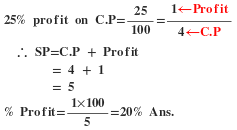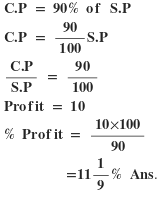# “The Knowledge Library”

#### Knowledge for All, without Barriers…

##### An Initiative by: Kausik Chakraborty.
09/02/2023 12:25 PM

# “The Knowledge Library”

Knowledge for All, without Barriers…

# “The Knowledge Library”

Knowledge for All, without Barriers……….

# Profit & Loss Part-1

## Marked price(M.P):The normal price of the thing without any discount.

Cost price(C.P): The price at which an article is purchased is called its cost price.

Selling price(S.P): The price at which an article is sold is called its selling price.

Profit or Gain: If S.P>C.P, The seller is said to have a Profit or Gain.

Loss: If S.P<C.P, The seller is said to have a Loss.

Concept: Discount% always calculated on marked price. profit% and loss% always calculate on Cost Price(C.P)

### Profit And Loss Formulas and tricks

Profit or Gain= S.P – C.P

Loss = C.P – S.P

%Profit or Gain = (Profit or Gain×100)/C.P

%Loss = (Loss × 100)/C.P

Concept:

Gain or Profit of x% can be represented as (100+x)/100

Loss of x% can be represented as (100-x)/100

If C.P and Profit% or Gain% given then

S.P=[C.P×(100+Gain%)]/100

If C.P and Loss’s% given then

S.P=[C.P×(100-Loss%)]/100

If S.P and Profit% or Gain% given then

C.P=(S.P×100)/(100+Gain%)

If S.P and Loss’s% given then

C.P=(S.P×100)/(100-Loss%)

Profit and Loss Formulas and important Questions

For Example:

(Q1)A man buys a cycle for Rs. 1400 and sells it at a loss of 15%. What is the selling price of the cycle?

(a)Rs 1202 (b)Rs 1190 (c)Rs 1160 (d)Rs 1000

Solution:

=>1400 × (100-15)/100 = S.P

=> 1400 × (85/100) = S.P

S.P = Rs. 1190 Ans.

(Q2)A man buys a cycle for Rs. 1400 and sells it at a profit of 20%. What is the selling price of the cycle?

(a)Rs 1500 (b)Rs 1560 (c)Rs 1580 (d)Rs 1680

Solution:

=> 1400 × (100+20)/100 = S.P

=> 1400 × (120/100) = S.P

S.P = Rs. 1680 Ans.

(Q3)There is 10% loss if an article is sold at Rs. 270, then the cost price of the article is.

(a)Rs 300 (b)Rs 270 (c)Rs 320 (d)Rs 250

Solution:

=> x × (100-10)/100=270

=> x × (90/100) = 270

∴ x = (270×100)/90

= Rs. 300 Ans.

BY Formula:

C.P=(S.P×100)/(100-Loss%)

C.P=(270×100)/(100-10)

=(270×100)/90

=Rs. 300 Ans.

(Q4)An item when sold for Rs. 1690 earned 30% profit on the cost price. Then the cost price is.

(a)Rs 507 (b)Rs 630 (c)Rs 1300 (d)Rs 130

Solution:

=>x × (100+30)/100=1690

=>x × (130/100)=1690

∴ x=(1690×100)/130

=Rs. 1300 Ans.

BY Formula:

C.P=(S.P×100)/(100+Profit%%)

C.P=(1690×100)/(100+30)

=(1690×100)/130

=Rs. 1300 Ans.

(Q5)A man buys an article for Rs. 27.50 and sells it for Rs. 28.60.Find his gain percent.

(a)5% (b)6% (c)4% (d)3%

Solution:C.P=Rs. 27.50

S.P=Rs. 28.60

Gain=S.P-C.P

=Rs. 28.60-Rs. 27.50

=Rs. 1.10

∴ Gain%= (Profit or Gain×100)/C.P

= (1.10×100)/27.50

= 4% Ans.

(Q6)If a radio is purchased for Rs. 490 and sold for Rs. 465.50, find the loss percent.

(a)5% (b)6% (c)4% (d)3%

Solution:C.P=Rs. 490

S.P=Rs. 465.50

Loss=C.P-S.P

=Rs. 490-Rs. 465.50

=Rs. 24.50

∴ Loss%=(Loss×100)/C.P

=(24.50×100)/490

=5% Ans.

(Q7)A fan is listed at Rs. 150 and a discount of 20% are given. Then the selling price is.

(a)Rs 180 (b)Rs 150 (c)Rs 120 (d)Rs 110

Solution:M.P=Rs. 150 Discount%=20%

Discount=150×(20/100)

=Rs. 30

S.P=M.P – Discount

=Rs. 150 – Rs. 30

=Rs 120 Ans.

Another way:

=>150 × (100-20)/100 = S.P

=>150 × (80/100) = S.P

S.P= Rs 120 Ans.

(Q8)If a Book costs Rs. 64 after 20% discount is allowed. What was its original price(i.e M.P) in Rs. ?

(a)Rs 80 (b)Rs 50 (c)Rs 75 (d)Rs 85

Solution:

=>x × (100-20)/100=64

=>x × (80/100)=64

∴ x= (64×100)/80

= Rs 80 Ans.

(Q9)A merchant purchases a table for Rs 450 and fixes its list price in such a way that after allowing a discount of 10%, he earns a profit of 20%. Then the list price of the table is.

(a)Rs 650 (b)Rs 700 (c)Rs 550 (d)Rs 600

Solution:C.P=Rs 450

Discount=10% and Profit=20%

M.P=?

=>450 × (100+20)/100 = S.P

=>450 × (120/100) = S.P

S.P= Rs 540

=>x × (100-10)/100 = 540

=>x × (90/100) = 540

∴ x = (540×100)/90

=Rs 600 Ans.

(Q10)Mohan purchased a machine for Rs 80,000 and spent Rs 5000 on repair and Rs 1000 on transport and sold it with 25% profit. At what price did he sell the machine?

(a)Rs 107500 (b)Rs 105700 (c)Rs 107550 (d)Rs 117600

Solution:Total cost price = 80,000+5000+1000

= Rs 86,000

S.P = 86000 × (100+25)/100

= 86000 × (125)/100

= Rs 107500 Ans.

(Q11)An Egg seller buys 240 egg for Rs. 600.Some of these eggs are bad and are thrown away.He sells the remaining egg at Rs. 3.50 each and makes a profit of 198. The percent of an egg thrown away are.

(a)6% (b)8% (c)5% (d)7%

Solution:C.P of an egg= 600/240

= Rs. 2.5

profit= Rs 198

Total S.P= Profit+C.P     [where Profit or Gain= S.P-C.P ]

= 198+600= Rs 798

Number of egg sold= 798/3.5

= 228

∴ Bad egg= 240 – 228 = 12

Now,

Required percentage= (12×100)/240

= 5% Ans.

(Q12)A shopkeeper bought 30Kg of rice at the rate of Rs. 70 per kg and 20 Kg of rice at a rate of Rs. 70.75 per Kg. If he mixed the two brands of rice and sold the mixture at Rs. 80.50 per Kg, his gain is.

(a)Rs 450 (b)Rs 510 (c)Rs 525 (d)Rs 485

Solution:

Gain= S.P – C.P

= 4025 – 3515 = Rs 510 Ans.

(Q13)A profit of 12% is made when a mobile phone is sold at Rs P and there is 4% loss when the phone is sold at Rs Q. Then Q:P is.

(a)1:1 (b)4:5 (c)6:7 (d)3:1

Solution:

=>x × (100+12)/100 = P

=>x × (112/100)= P

112x = 100P

P= 112x/100

Now,

=>x × (100-4)/100= Q

=>x × (96/100)= Q

=>96x = 100Q

Q= 96x/100

Now,

Q/P= (96x/100)/(112x/100)

= 6/7

Q:P= 6:7 Ans.

(Q14)If the ratio of cost price to selling price is 10:11, Then the rate of a percent of profit is.

(a)1.1% (b)0.1% (c)10% (d)1%

Solution:Let C.P= 10x

S.P= 11x

Profit= 11x – 10x = x

∴ % Profit=(x × 100)/10x

=10% Ans.

(Q15)An article is sold at 5% profit. The ratio of selling price and cost price will be.

(a)1:5 (b)20:21 (c)21:20 (d)5:1

Solution:

=>x ×(100+5)/100= S.P

S.P= 105x/100

Now,

S.P/C.P= (105x/100)/x

= 21/20

∴ S.P:C.P= 21:20 Ans.

(16)A store sells watch for a profit of 25% of the cost. Then the percentage of profit against selling price is.

(a)22% (b)20% (c)18% (d)15%
Solution:-Second Method:-(17)By selling an article, a man makes a profit of 25% of its selling price. His profit percent is.
(a)20% (b)25% (c)16 ²/3 %  (d)33 ¹/3 %

Solution:-(18)If the cost price is 90% of the selling price, what is the profit percent?
(a)4% (b)4.75% (c)5% (d)11 ¹/3 %
Solution:-